2. ELECTROSTATIC POTENTIAL AND CAPACITANCE

Q.1. What do you mean by saying that electrostatic field in conservative in nature ?

Ans ⇒ An electric field is said to be conservative if :

(i) Line integral of electric field between two given points is independent of the nature of path followed.
(ii) Line integral of electric field along a closed path is zero.

Q.2. What are properties of equipotential surfaces ?

Ans ⇒ Following are the properties of equipotential surfaces :

(i) No work is done in moving a test charge over an equipotential surface from one point to another.
(ii) The electric field is always perpendicular to the equipotential surface.
(iii) The equipotential surfaces help us to distinguish regions of strong field from those of weak field.
(iv) The equipotential surfaces tell us the direction of electric field.
(v) No two equipotential surfaces intersect each other.

Q.3. Define polarisation.

Ans ⇒ It is defined as the process of stretching of dielectric atoms due to the displacement of charges in the atoms under the action of applied electric field. This stretching of atoms continues till the restoring force becomes just equal and opposite to the force exerted by the applied electric field on the charges. Thus C.G. of +ve nucleus does not coincide with the C.G. of electrons that are – vely charged due to this stretching and the atom thus acquires a dipole moment.

Q.4. Write an expression for capacitance of a parallel plate capacitor.
(i) When a dielectric medium of dielectric constant K between its plate.
(ii) When a slab of thickness t1 t < d1 d = separation between plates and dielectric constant K is introduced between the plates of the capacitor.

Ans ⇒ Capacitance of a parallel plate capacitor with air as the medium between two plates of are A lying at a distance d part is given by

C = ε0A/d

Let a dielectric slab of thickness t be put between the plates such that t < d. Due to polarisation, electric field will reduce from E0 to E.

Potential difference across the capacitor is given by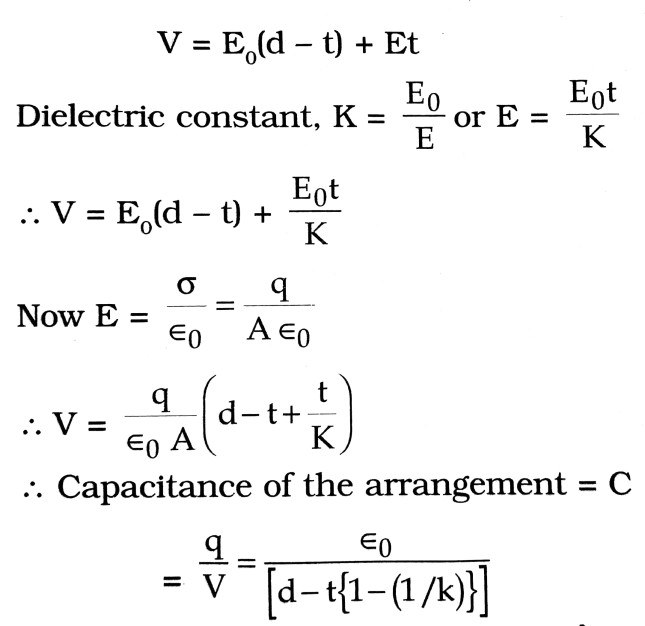Q.5. Define the term ‘electrostatic potential’. Give the dependence of electrostatic potential due to a small electric dipole at a far off point lying on (i) the axial line and (ii) equatorial line.

Ans ⇒ Electrostatic potential at any point is the amount of work done in bringing a unit positive charge from infinity to that point.
At any far off point lying on the axial line of a dipole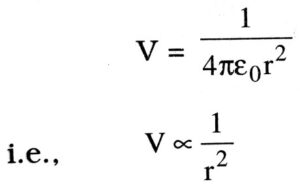At any equatorial point, V = 0.

Q.6. Briefly explain the principle of a capacitor. Obtain the expression for the capacitance of a parallel plate capacitor.

Ans ⇒ A capacitor works on the principle that the capacitance of a conductor increases when an earthed corrected conductor is placed near it.
A parallel plate capacitor consists of two (large) parallel conducting plates, separated by a (small) distance (Fig.). The two plates of the capacitor have equal but opposite charge densities of magnitude δ. The electric field at any point between the plates is E
= δ/ε0.

Let d be the distance between the plates. A be the area of each plate and Q be the magnitude of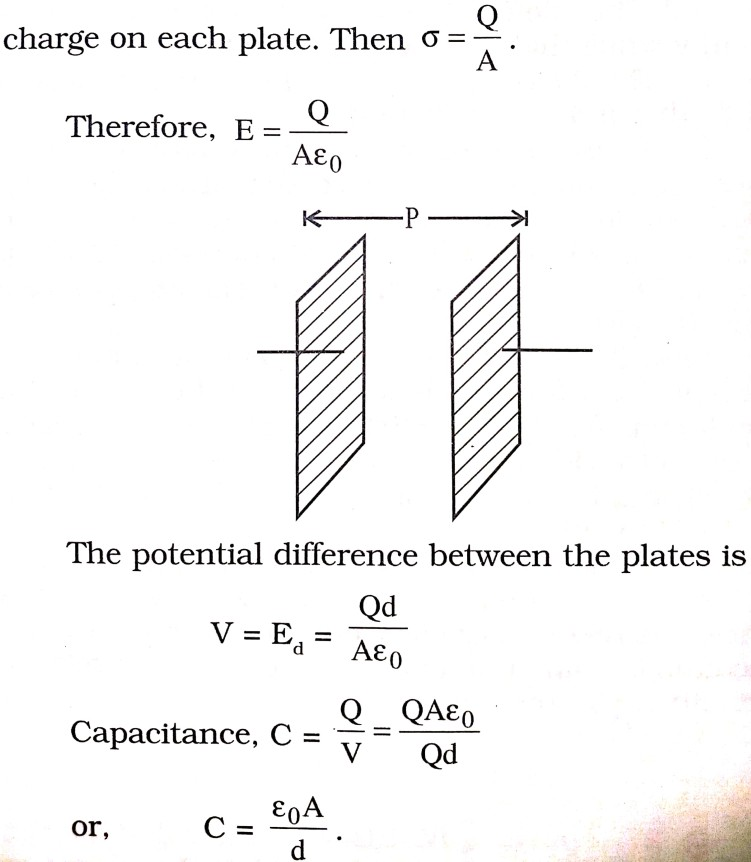Q.7. Two charged conducting spheres of radii a and b are connected to each other by a wire. What is the ratio of electric fields at the surfaces of the two spheres ? Use the result obtained to explain why charge density on the sharp and pointed ends of a conductor is higher than on its flattened portions.

Ans ⇒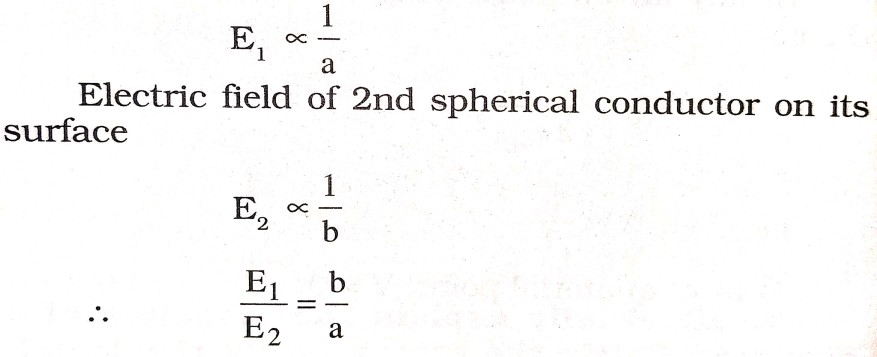Since a flat portion may be equated as a spherical surface of large radius (i.e., lower charge density, as charge density = charge/area) and a pointed portion to one of small radius (i.e., highest charge density).

Q.8. What are the differences between conductor and dielectrics ?

Ans ⇒ (i) Conductors have a large number of free electrons while dielectrics have practically no free electrons.

(ii) When a conductor is placed in an external electric field, there is no electric field inside the conductor. However, when a dielectric is placed in an electric field, its molecules are polarised. The effect of this polarisation is to weaken the applied electric field within the dielectric.

(iii) The dielectric constant of conductors is infinity while that of dielectric is finite.

(iv) The dielectric strength of conductors is zero while that a dielectric is finite.

(v) There is no limit to the current that a conductor can carry, provided that it can be keep cool enough. However, there is a limit to the electric flux that a dielectric will carry without breaking down.

Q.9. Define dielectric strength and relative permittivity.

Ans ⇒ Dielectric strength : It is that intensity of electric field for which a dielectric becomes non conductor. When the electric field intensity is greater than dielectric strength the atoms or molecules of dielectric are ionised. For air the dielectric strength is 3 x 106 vm-1.

Relative permittivity : The ratio of permittivity of any medium and the permittivity of free space is known as relative permittivity. If the permittivity of a medium is ε and that of free space is ε0 then relative permittivity of the medium is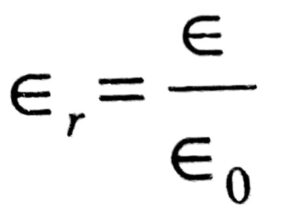Q.10. Applying Biot Savart’s law deduce the expression for the magnetic field at the centre of a semicircular wire loop of andius R carrying a current I.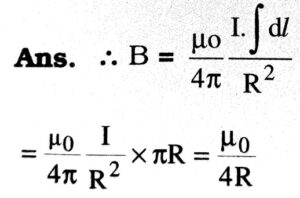## Class 12th physics Subjective question in English

 S.N Physics Short Type Question English Medium 1. ELECTRIC CHARGES AND FIELDS 2. LECTROSTATIC POTENTIAL AND CAPACITANCE 3. CURRENT ELECTRICITY 4. MOVING CHARGES AND MAGNETISM 5. MAGNETISM AND MATTER 6. ELECTROMAGNETIC INDUCTION 7. ALTERNATING CURRENT 8. ELECTROMAGNETIC WAVES 9. RAY OPTICS AND OPTICAL INSTRUMENTS 10. WAVE OPTICS 11. DUAL NATURE OF MATTER AND RADIATION 12. ATOMS 13. NUCLEI 14. SEMI CONDUCTOR ELECTRONICS 15. COMMUNICATION SYSTEMS